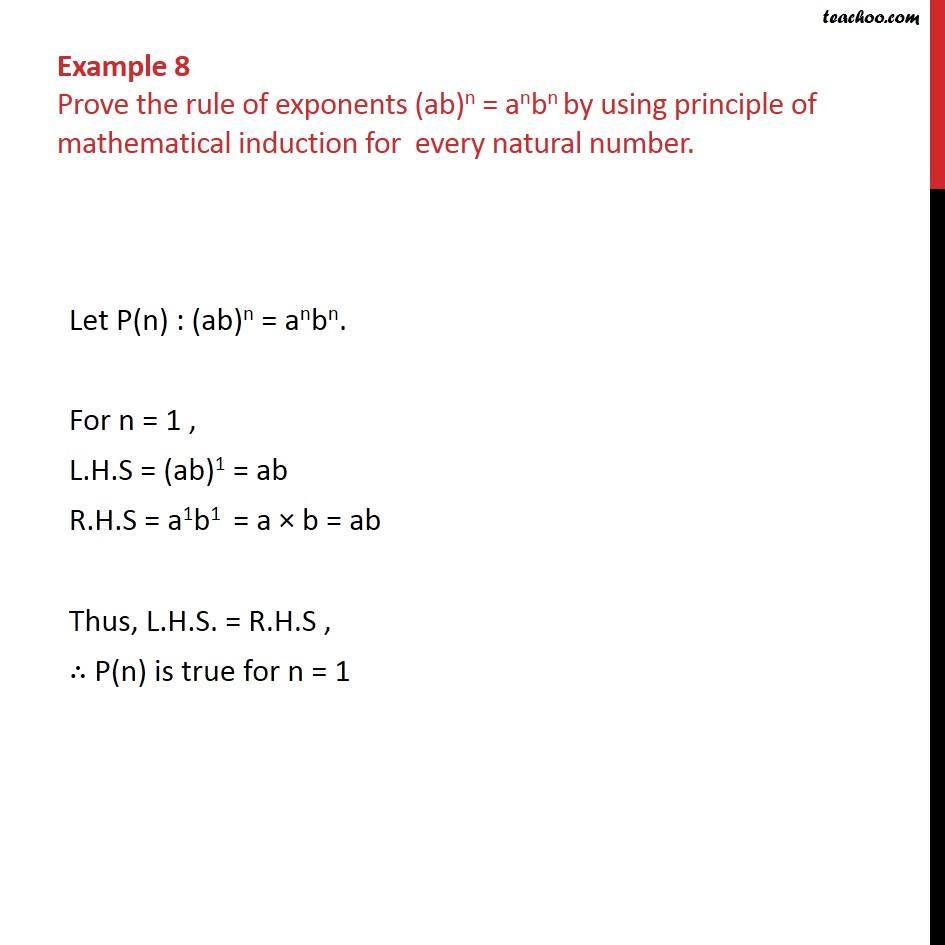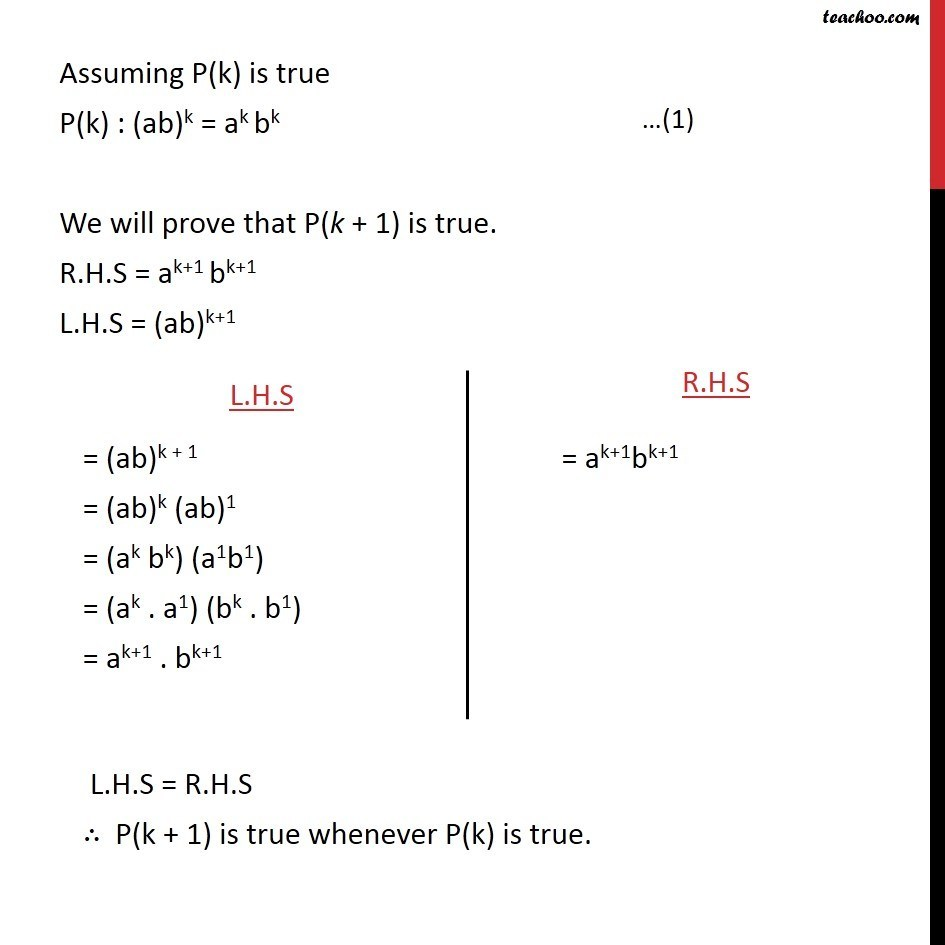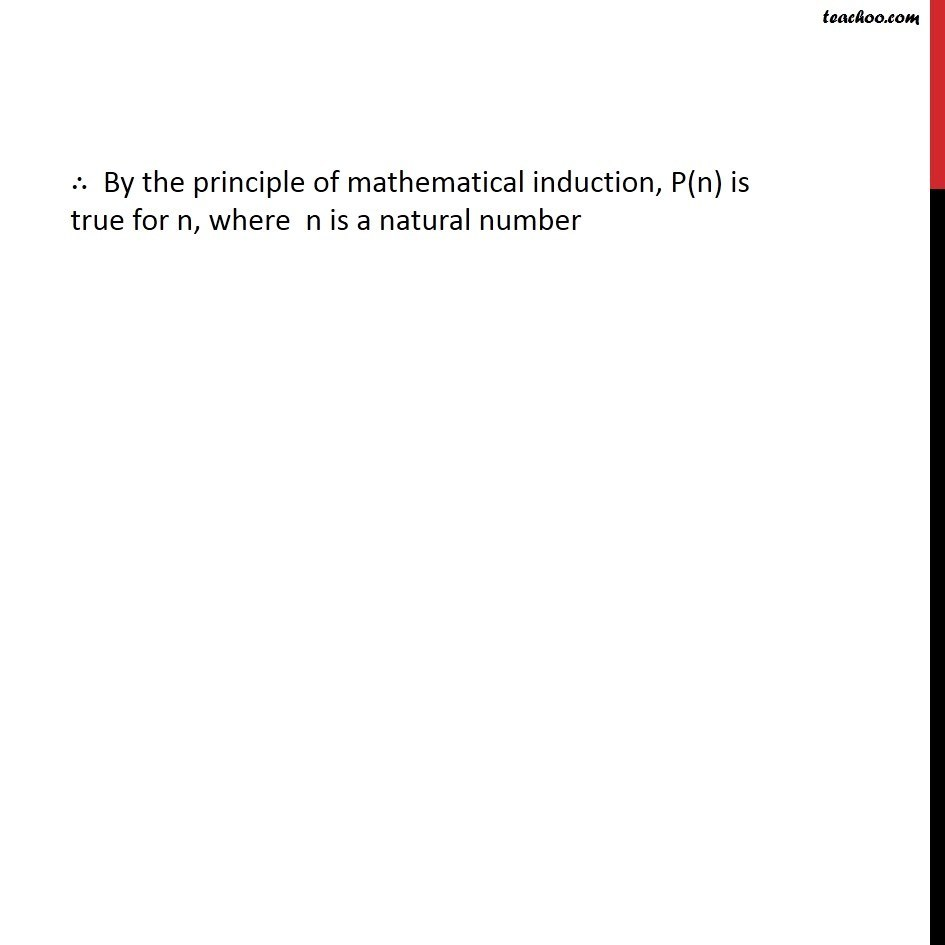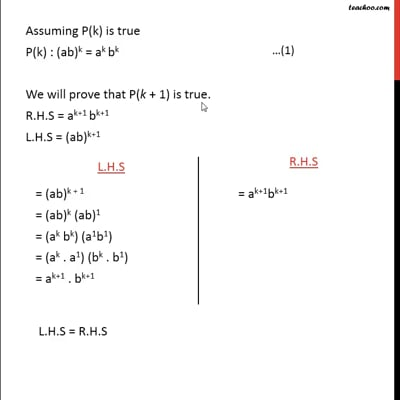Examples

Chapter 4 Class 11 Mathematical Induction
Serial order wiseThis video is only available for Teachoo black users

Introducing your new favourite teacher - Teachoo Black, at only ₹83 per month

### Transcript

Example 8 Prove the rule of exponents (ab)n = anbn by using principle of mathematical induction for every natural number. Let P(n) : (ab)n = anbn. For n = 1 , L.H.S = (ab)1 = ab R.H.S = a1b1 = a b = ab Thus, L.H.S. = R.H.S , P(n) is true for n = 1 Assuming P(k) is true P(k) : (ab)k = ak bk We will prove that P(k + 1) is true. R.H.S = ak+1 bk+1 L.H.S = (ab)k+1 By the principle of mathematical induction, P(n) is true for n, where n is a natural number